The bandwidth of a measurement system is its most important figure of merit. As part of situational awareness, we want to verify the measurement system’s bandwidth is at least 2x higher than the DUT’s signal bandwidth. This is so we do not miss the important features of the signal or introduce measurement artifacts.

While we get the scope’s bandwidth from the vendor, as soon as we add a cable, probe, or amplifier to the scope, we decrease the system bandwidth. The new system bandwidth is as important to know as the scope’s bandwidth, but it is generally difficult to measure except in a calibration lab. We offer a simple method of evaluating the transfer function and system bandwidth of any probing system using a wide band noise source.

Measuring transfer functions

When we use a single figure of merit, like bandwidth, to describe a transfer function, we are making a lot of assumptions, like: the transfer function looks like a low pass filter, the passband region is flat, and the roll-off region is the transition from flat to a constant downward slope. We could use another figure of merit, like the filter order, to describe how fast the transfer function drops off with frequency. An example of the measured transfer function of a scope with minimal connections to the source, is shown in Figure 1.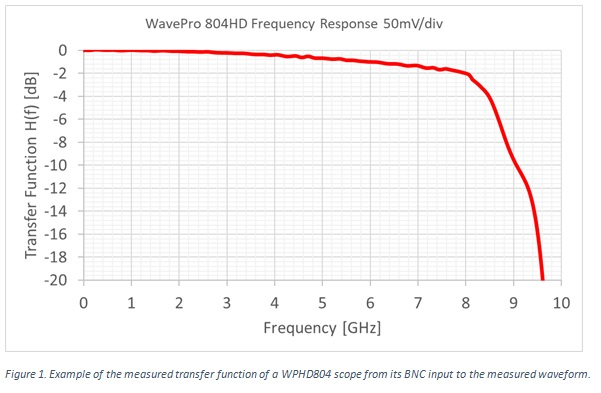There are a number of ways to measure the transfer function of a measurement system. Unfortunately, using a VNA is not one of the. We really want to be able to include the scope’s amplifier and whatever DSP equalization is built into the scope’s electronics, in addition to the passive cables and probes. Other than in a calibration lab, we can’t pull these pieces out and connect a VNA from the input to the output.

However, we could use a sine wave source, with a flat frequency response. We could sweep its frequency from 1 MHz to 10 GHz and measure the amplitude of the sine wave at various frequency steps. This requires a very flat, high bandwidth sine wave source.

We could use a very fast step signal as an input. If this is part of a 10 MHz clock, for example, its spectrum would be a comb pattern of peaks at odd multiples of 10 MHz. But, the amplitude of each harmonic drops off as 1/f, so there is worse signal to noise ratio (SNR) at higher frequency, where we are more interested in the transfer function roll off.

The method we introduce here uses a Noisecom NC1100 wide-band noise source with frequency components extending from 1 MHz to > 10 GHz. We measure this signal at the input to the Teledyne LeCroy WavePro HD 804 using the highest bandwidth connection we can, calculate its FFT to get the spectrum, and use this as the stimulus. Then we insert the cables and probes and measure the changed response.

On a log amplitude scale, the difference between the distorted spectrum and the reference spectrum is a measure of the transfer function of just the cable-probe system. This method not only gives us information about the probes and interconnects, but it also tells us how the scope responds to the measurement system, information which cannot be measured by a VNA alone.

Transfer Functions from a Noise Source

A wideband white noise source will have a power density relatively constant over frequency. Compared to square wave source, there will be higher signal power at high frequency, offering a constant SNR across the frequency range.

In effect, a wide-band noise source is probing all frequencies at the same time. It’s only when viewed in the frequency domain that we see all the frequency components.

The process we use to measure the transfer function of the measurement system can be applied to any measurement system:

1. Using the fastest sampling rate, measure the voltage noise directly from the source and calculate the FFT. This gives the highest frequency limit to the FFT.
2. Select a time base which defines the bin size for reasonable resolution.
3. Average the FFT 300 times to reduce the inherent noise and get a smoother signal
4. Store this as the reference received signal
5. Plug in the probe-cable system under test, and measure its spectrum with the same settings
6. Subtract the reference spectrum from the DUT spectrum, on a log scale and the difference is the transfer function change from the measurement system
7. Use this approach to explore the transfer function of your systems.

The first step is to characterize the noise source. The highest sample rate for the scope used in this example is 20 GSamples per second. This results in a highest frequency in the FFT of 10 GHz, limited by the Nyquist sampling rate. The time base is 1 usec full scale, or 100 nsec/div. This results in a bin size, or resolution in the spectrum, of 1 MHz. This is the base set up.

The FFT is calculated using a von Hann window function. The time domain signal and FFT are shown in Figure 2 for the case of a single FFT sweep and then after 300 consecutive FFT sweeps are averaged. The averaged spectrum is the reference spectrum.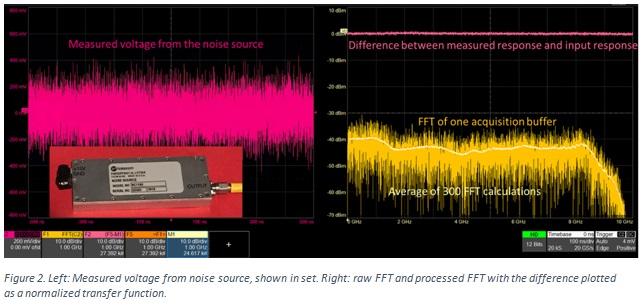If we assume the scope’s intrinsic transfer function is flat, this measurement of the noise source spectrum suggests the noise source is not a perfectly flat frequency response. It varies about +/- 3 dB in amplitude.  But it has significant energy up to very high frequency. The role-off in the spectrum above 8 GHz is a direct measure of the scope’s rated bandwidth of 8 GHz. This measurement is not possible with a VNA.

The upper plot is the calculated, normalized transfer function of the measured spectrum minus the reference spectrum. In this example, it is a flat 0 dBm, since we are looking at the reference noise source compared to itself.

Many scopes offer a front-end filter to reduce the measurement bandwidth. When the signal’s bandwidth is low, reducing the scope bandwidth will reduce the high frequency noise, where there is no signal content, increasing the SNR of the measurement. Figure 3 is an example of the transfer function of the system with the scope bandwidth set to 4 GHz.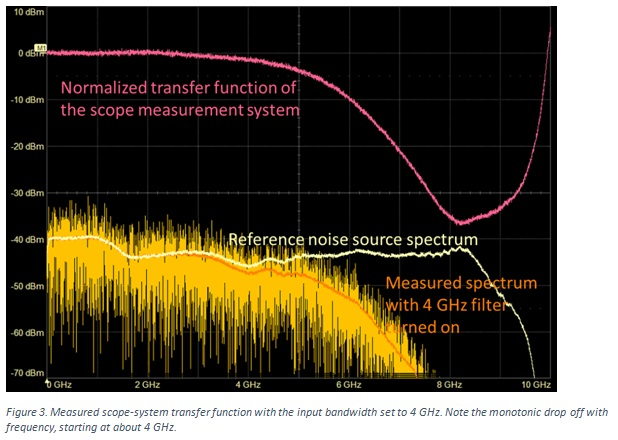As examples of using this technique to gain insight into the properties of common measurement applications, we look at:

• The input coupling settings of the scope
• The impact of different cables and tips
• The impact of a 10x passive probes under the best case and typical conditions.

Bandwidth of input coupling settings

The highest bandwidth measurement is when the input coupling is set to 50 Ohms. This will use the highest bandwidth of the scope and terminate the scope-end of the cable to prevent reflections.  When we want the highest bandwidth of the scope, we always should use the 50 Ohm coupling setting.

When the coupling is set for 1 Meg input, either DC or AC coupled, the bandwidth drops to about 1.2 GHz. This is due to a different setting for the scope amplifier on the 1 Meg input setting. This response is shown in Figure 4.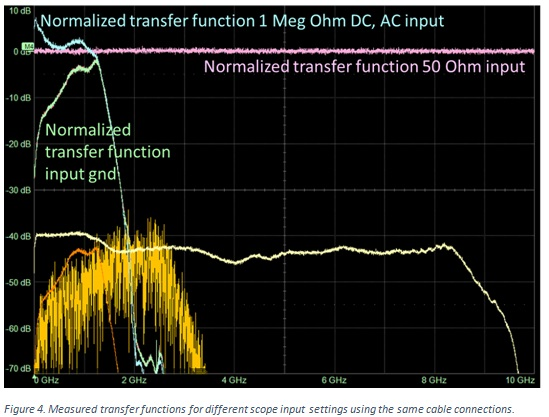The last setting for the input coupling is grounding the input to the scope’s amplifier. This will connect the input to the scope’s amplifier to an internal ground. It will not short the DUT which would still be connected to the front BNC connector to the scope.

It is important to note that there is no such thing as a short above 100 MHz. This internal short behaves like a small inductor in close proximity to the input pin. The near-field electric and magnetic field coupling between the input pin and the amplifier input is not zero. As shown in the measurement above, there is almost -3 dB coupling from 700 MHz to 1.2 GHz between the amplifier input and the BNC input. This is when the input to the amplifier is nominally grounded.

When the input termination is set for 1 Meg Ohm (which is typically used so we don’t load the DUT down with a low DC resistance), the system bandwidth is reduced from 8 GHz to about 1.2 GHz with a very fast roll off.

How can we have a 6 dB gain in a passive probe system?

It is important to note that at low frequency, from 1 MHz to about 200 MHz, the response of the measurement system, with the coax cable and 1 Meg Ohm input resistance of the scope shows a gain of 6 dB. This is a 2x higher signal amplitude. The root cause of this behavior is a good test of how well you understand what is actually being measured by the scope.

Every voltage source has, internally, some Thevenin voltage amplitude and Thevenin source resistance. In the case of the Noisecom NC1100 device, the source resistance is 50 Ohms. When we measure the voltage in the scope with 50 Ohms input impedance, we have created a voltage divider with the 50 Ohm source resistance. This means the voltage the scope measures is NOT the internal Thevenin voltage of the source, but half of this voltage. This is the normalized signal we measure: the voltage launched into a 50 Ohm load.

When we set the input impedance of the scope to 1 Meg, the voltage launched into the cable from the source hasn’t changed. This signal hits the 1 Meg resistance and nearly 100% of it reflects and heads back to the source, where this reflected wave is terminated by the source series Thevenin resistance.

At the scope, we measure two waves, the incident wave and the reflected wave. This results in a measurement of twice the DUT voltage, with a 1 Meg termination, compared with a 50 Ohm termination. A factor of 2 is 6 dB higher.

This behavior is a direct measure of how the scope interacts with the cable-probe measurement system. It cannot be measured with a VNA, but must be measured by the scope itself.

Given the measurement bandwidth drop off with a 1 Meg input resistance setting on the scope, how do we engineer a measurement with a high bandwidth, but also high impedance at DC?

High Impedance AND High Bandwidth

The way of getting the best of both conditions is using an active probe. An example of the measured transfer function of a rail probe RP4030 is shown in Figure 5. The rated bandwidth is 4 GHz, and matches the measured transfer function very well.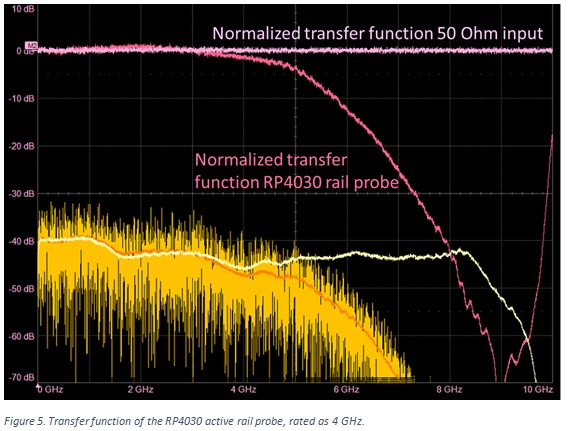The input impedance of the rail probe is 50 k Ohms at low frequency, but drops off to 50 Ohms above 100 kHz where reflections might be a concern.

Bandwidth of Cables and Tips

The most common sort of cable available in every lab is an RG58 coax cable. There is always some attenuation in these cables. Figure 6 shows the transfer function of four cable configurations:

1. A 1 m long VNA quality 50 Ohm coax cable
2. A 1 m long RG174 coax cable costing \$7 per cable assembly
3. A 2 m long RG58 cable costing \$10 per cable assembly
4. A 1 m long RG174 cable costing \$2 per cable assembly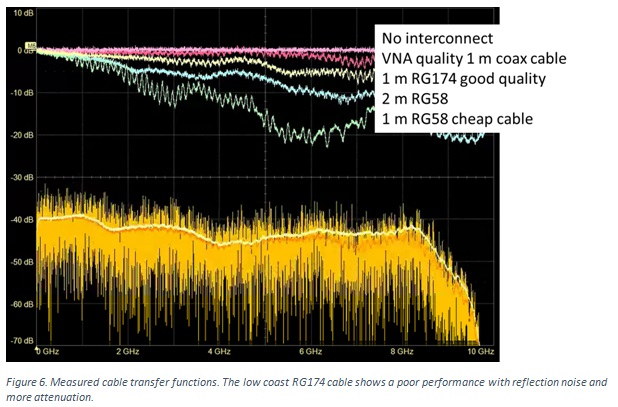These simple measurements offer a few rules of thumb. A good quality 2 m RG58 cable has a -3 dB bandwidth of about 1.5 GHz. A good quality 1 m long RG174 cable has a -3 dB bandwidth of about 5 GHz. Both of these cables have the same BNC connectors on their ends. The difference is in the cable attenuation and the length. Watch out for cheap RG58 cables if you expect to do any signal integrity measurements. The measured performance of this 1 m cheap RG174 cable shows significant ripple from reflection noise from the BNC connectors. Generally, at this price point, the connector terminations are often not very good.

When the tip is not coaxial, but pulled apart using a mini-grabber, the bandwidth of the measurement system immediately drops. Figure 7 shows the measured bandwidth with a large tip loop inductance and when the wires are twisted together.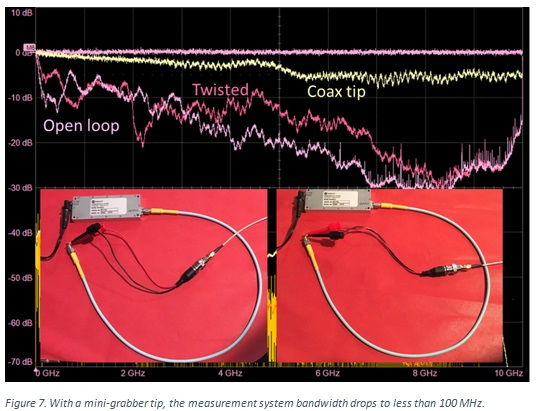While twisting the wires at the tip is a good habit, don’t expect this trick to increase the bandwidth very much. At best, it might be 200 MHz, and then strongly dependent on the details. The way to achieve high bandwidth probing is to make the tip look as much like a 50 Ohm coax as possible.

As soon as we spread the signal and return paths apart when we use a mini-grabber tip, we introduce an impedance discontinuity. We can think of this as adding reflections at the tip, reflecting the higher frequency components, acting like a low pass filter, or as a discrete inductance, generating an L/R low pass filter. Either way, the larger the tip loop inductance, the larger the impact on the measurement system bandwidth.

Bandwidth of the 10x Probe

The 10x probe is actually a complex probing system. Built into it is a low pass filter with a roll-off frequency of about 10 kHz, a parallel high-pass filter with a pole frequency at about 10 kHz, and a special, very lossy coax cable which absorbs and attenuates any high frequency reflections in the cable.

In order to use the 10x cable, 1 Meg coupling has to be used in the scope. This automatically drops the measurement system bandwidth to about 1.2 GHz due to the scope amplifier. To see the absolute highest bandwidth of the probe, the tip is replaced with a coax adaptor. This is very different from the typical 10x probe with a large loop inductance at the tip.

These two cases are compared in Figure 8.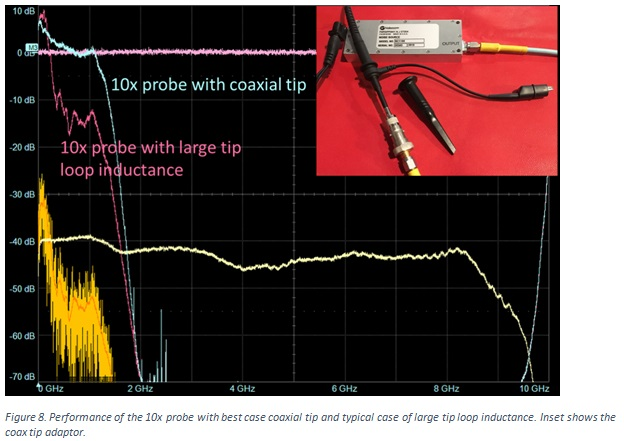Note that the transfer function of the 10x probe in both the coax tip and the large loop tip, show about 6 dB amplitude at low frequency. This is because the source impedance is 50 Ohms and the input impedance of the scope is 1 Meg, as described earlier.

As a general rule, unless special care is taken, assume the bandwidth of a 10x probe is about 100 MHz.

Conclusion

While the scope bandwidth is an important figure of merit to describe the highest frequency component the scope can measure, changing its input coupling, and adding cables and probes will decrease this measurement bandwidth.

Having a simple way of measuring not just the signal bandwidth but the entire transfer function into the scope is a valuable way of characterizing your measurement system. Knowing the transfer function of the measurement system, compared to the signal spectrum from your DUT, will give you the situational awareness to know what is real about your DUT’s signal and what might be a measurement artifact.